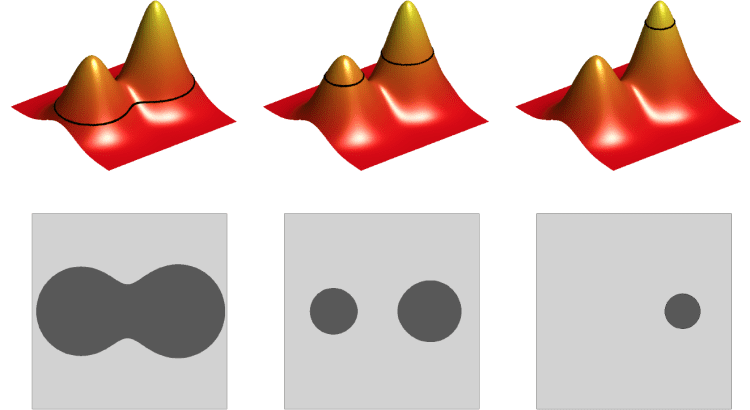# 4D tomography: walkthrough of my project - part 2After talking about motivation (see the first part and then part 3), I will now go into details with the mathematics foundations of the project. The novel tomography reconstruction algorithm I am contributing developing is based on a level set method approach.

#### Level set methods

A level set method is an elaborate, yet geometrically intuitive, framework to deal with a dynamic front. Imagine the problem of a 2D object changing in time. For instance, let's say we have a disk that stays still for a while, then expands in a "eigth shape" and then splits into disks that keep moving. After a while, a smaller disk originates from one of the previous two. In a situation like this, we would witness a topological change that is quite hard to parametrize (*). The intuitive idea behind level set methods is to model such situation in 3D, including time as a spatial dimension. The dynamic 2D object will then "build" a continuous surface. You can observe the case I depicted in the following video (**).

On the left, you can observe the 2D dynamic object changing in time. On the right, the level set surface is built accordingly.

Level set methods were originally developed in the 1980s by mathematicians Stanley Osher and James Sethian. The motivating application was (still is) computer graphics, where problems like the one I described above are frequent, for instance, in reproducing animation of fluids, where topological changes are routine.

As Osher put it, "when a catastrophe in the movies should look realistic, Hollywood calls for the mathematicians".

#### Our model

Level set methods were applied to several inverse problems and you can learn more about it from this nice survey (2004). In this case, we model the X-ray attenuation (that is the unknown we want to recover) as , where  is a cut-off function we choose (I will explain how in the next post) and  is the minimizer of the following Tikhonov-like functional:

(1)

For someone who works in iterative reconstruction algorithms, this looks familiar (***). The main difference is the presence of the function . Here  is the regularization parameter, that has the task to balance the two norms. Now, through Gateaux differentiation (§), one can see that solving this minimization problem is equivalent to finding the limit solution  of the evolution equation:

(2) 

In this sense, this is a level set method, since equation (2) recalls an evolution equation of a level set method. Anyway, I approach the numerical solution of the problem by the formulation (1) and apply gradient descent methods.

In the next post I will explain who  is and how we choose it. Also, I will show some published results to present a comparison with well-known methods in the case of undersampled data.

(*) If you had the instinct of running away at "topological change", don't panic. In simpler words, the trouble is at the instant when the disk splits in two. Such geometric change is tricky.

(**) The phantom was created by postgrad student Esa Niemi, the video was assembled by master student Topias Rusanen. Please mention the authors if you embed the video somewhere.

(***) For those who do not, this is a classical regularization problem formulation.

(§) For details, see Niemi et al. and Kolehmainen et al..#### Paola Elefante

Digital Scaling Project Manager at Plan International. Proud mother & wife. Shameless nerd&geek. Feminist. Undercover gourmet.

This site uses Akismet to reduce spam. Learn how your comment data is processed.